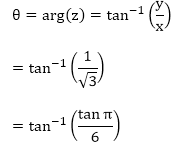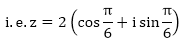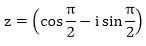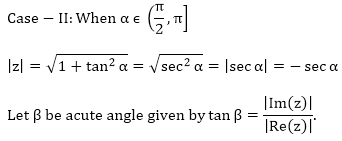×#### Thank you for registering.

One of our academic counsellors will contact you within 1 working day.

Click to Chat

1800-1023-196

+91-120-4616500

CART 0

• 0

MY CART (5)

Use Coupon: CART20 and get 20% off on all online Study Material

ITEM
DETAILS
MRP
DISCOUNT
FINAL PRICE
Total Price: Rs.

There are no items in this cart.
Continue Shopping• Complete JEE Main/Advanced Course and Test Series
• OFFERED PRICE: Rs. 15,900
• View Details

```Chapter 13: Complex Numbers – Exercise 13.4

Complex Numbers – Exercise 13.4 – Q.1(i)

The polar form of a complex number z = x + iy, is given by z = |z| (cos⁡ θ + i sin ⁡θ)

where,Let z = 1 + i∵ x, y > 0, so θ lies in first quadrant

Now,Complex Numbers – Exercise 13.4 – Q.1(ii)

The polar form of a complex number z = x + iy, is givenby z = |z| (cos ⁡θ + i sin ⁡θ)

Where,= 2

∵ x = √3. 0 & y = 1 > 0,

∴ θ  lies in first quadrant

Hence= tan-1⁡  (∵ tan-1)⁡ (tan⁡ x) = x)

polar form is given by z = |z|(cos ⁡θ + i sin ⁡θ)Complex Numbers – Exercise 13.4 – Q.1(iii)Complex Numbers – Exercise 13.4 – Q.1(iv)Polar Form, z = r (cos ⁡θ + i sin ⁡θ)Complex Numbers – Exercise 13.4 – Q.1(v)Complex Numbers – Exercise 13.4 – Q.1(vi)

The polar form of a  complex number z = x + iy, is given by z = |z| (cos ⁡θ + i sin ⁡θ)

where,Complex Numbers – Exercise 13.4 – Q.1(vii)

The polar form of a complex number z = x + iy, is given by z = |z| (cos ⁡θ + i sin ⁡θ)

Where,Here z is  already inpolar formComplex Numbers – Exercise 13.4 – Q.1(viii)

The polar form of a complex number z = x + iy, is given by z = |z| (cos ⁡θ + i sin ⁡θ)

Where,= 8

Here x = – 4 < 0 & y = 4R3 > 0, ∴ θ lies in quadrant IIComplex Numbers – Exercise 13.4 – Q.2

z = (i25)3 = (i)3 = – i

|z| = 1,Complex Numbers – Exercise 13.4 – Q.3(i)

Let z = 1 + i tan⁡ α

tan⁡ α is periodic fun function with period π.tan ⁡β = |tan ⁡α| = tan ⁡α

⟹ β = α

As z is represented by a point in first quadrant.

∴ arg⁡(z) = β = α

So polar form of z is sec⁡α (cos ⁡α + i sin ⁡α)tan⁡ β = |tan ⁡α| = – tan⁡ α = tan⁡(π – α)

⟹ β = π – α

As z is represented by a point in fourth quadrant.

∴ arg⁡(z) = – β = α – π.

So polar form of z is-sec⁡α (cos⁡ (α – π) + i sin⁡ (α – π)).

```### Course Features

• 728 Video Lectures
• Revision Notes
• Previous Year Papers
• Mind Map
• Study Planner
• NCERT Solutions
• Discussion Forum
• Test paper with Video Solution